• 一、一元线性回归 一元线性回归：找离散的点总体上“最逼近”那条直线。同理， 二元线性回归：找离散的点在空间中总体上“最逼近”的那个平面。 一元线性回归 #散点 数据 x1=np.arange(1,10,1) y1=np.array...
一、一元线性回归

一元线性回归：找离散的点总体上“最逼近”那条直线。同理，

二元线性回归：找离散的点在空间中总体上“最逼近”的那个平面。

一元线性回归

#散点 数据
x1=np.arange(1,10,1)
y1=np.array([1,3,3,3,5,6,7,8,10])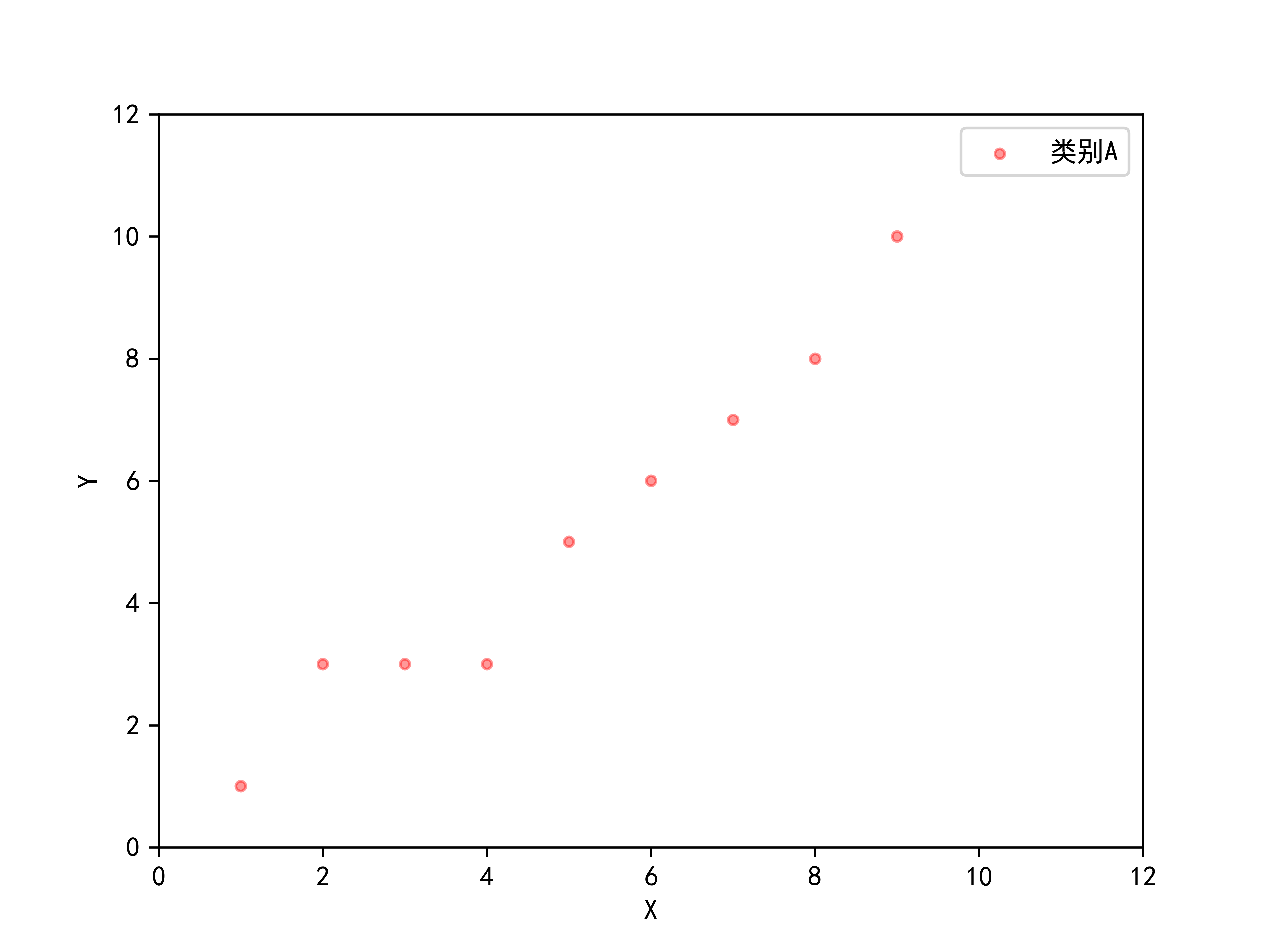我们设要拟合的直线为: y = ax + b，求出参数a,b; 已知的数据点为: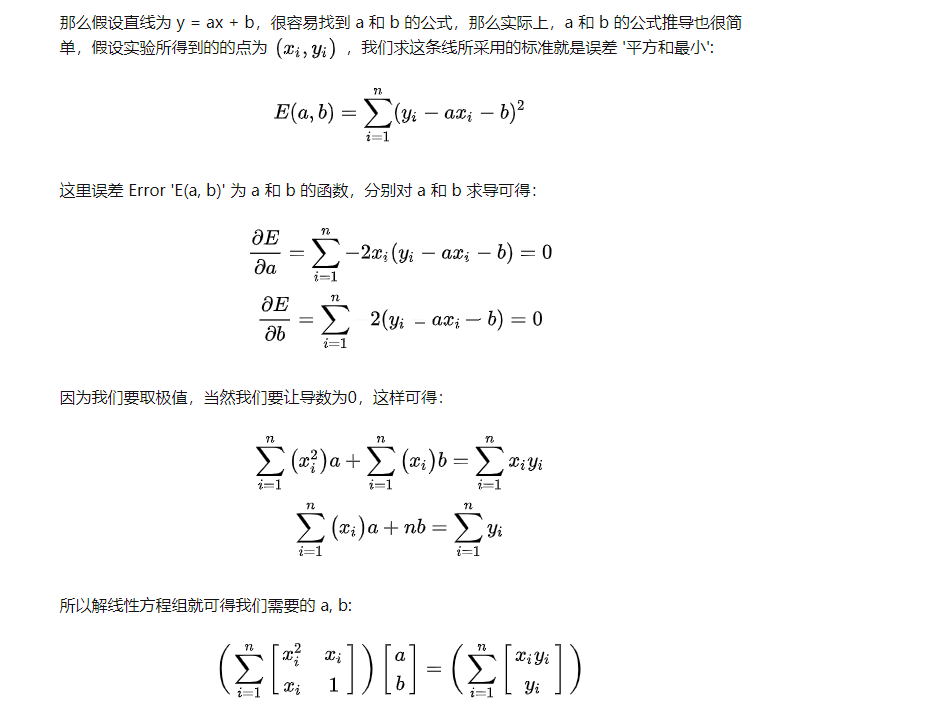代码实现：

# 2020.01.06  By yangbocsu
import numpy as np
import matplotlib.pyplot as plt
import math

#matplotlib画图中中文显示会有问题，需要这两行设置默认字体
plt.rcParams['font.sans-serif']=['SimHei']
plt.rcParams['axes.unicode_minus'] = False

#画的坐标轴并设置轴标签x，y
plt.xlabel('X')
plt.ylabel('Y')
plt.xlim(xmax=12,xmin=0)
plt.ylim(ymax=12,ymin=0)

#散点图数据
x1=np.arange(1,10,1)
y1=np.array([1,3,3,3,5,6,7,8,10])

plt.scatter(x1, y1, marker = 'o', alpha=0.4,color="red", label='离散点')
plt.legend() #label='类别A' 图中显示
plt.title('一元线性回归')#图的标题

#拟合的直线为:y = ax + b，求出参数a,b
A = np.empty([2, 2])
B = np.empty([2, 1])
for i in range(len(x1)):
A+=x1[i]*x1[i]
A+=x1[i]
A = A
A+=1
B+=x1[i]*y1[i]
B+=y1[i]

ab_para=np.dot(np.linalg.inv(A),B)
a  = ab_para
b  = ab_para

print("a=",str(a))
print("b=",str(b))

x2 = np.arange(0,12,1)
y2 = a*x2 + b

plt.plot(x2,y2,label="拟合直线")
plt.legend()

plt.savefig(r'C:\Users\97942\Desktop\hw1_data\线性回归.png', dpi=600)
plt.show()



a= 1.0568055403642536
b= -0.2049425235228064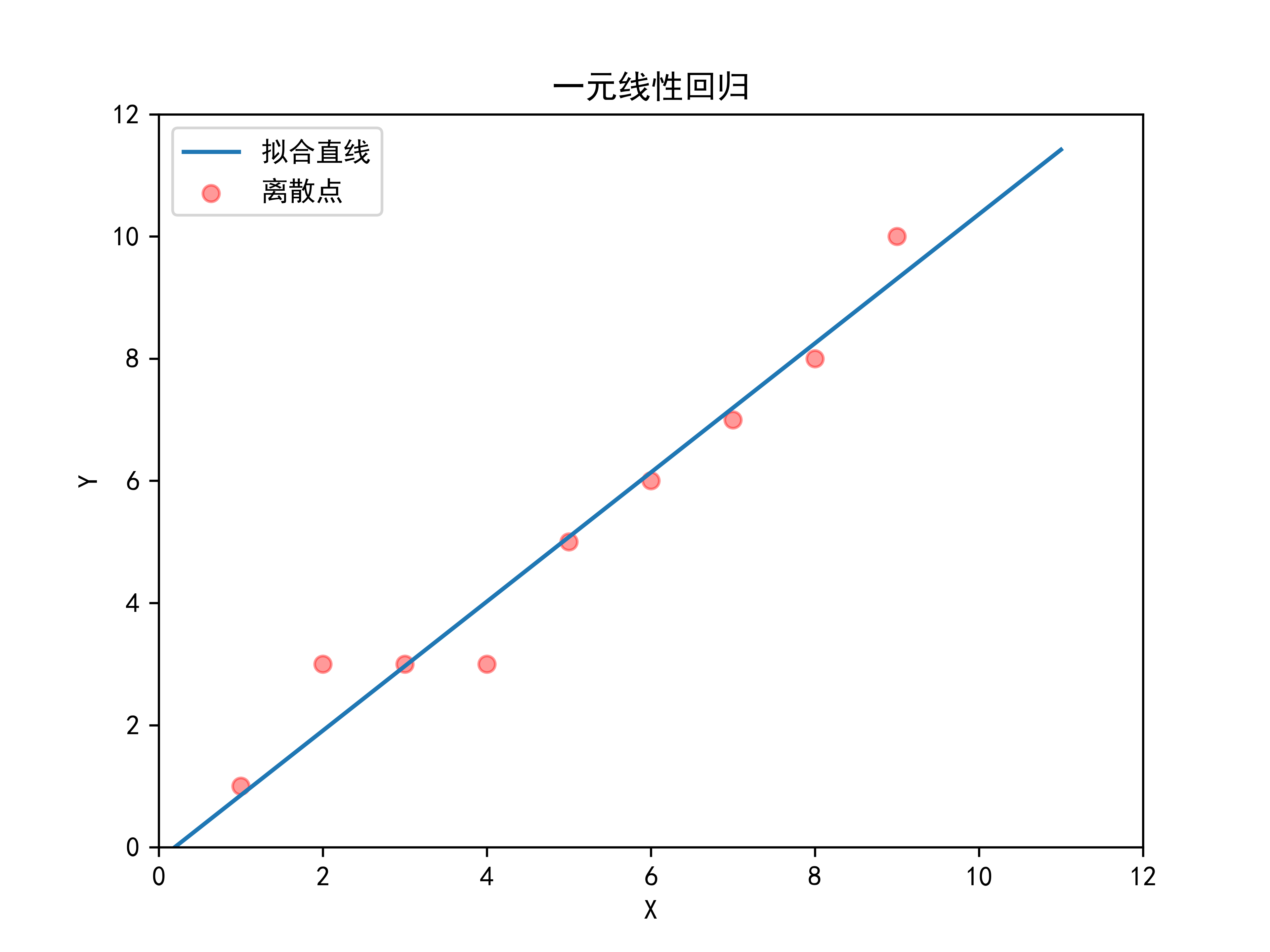进阶版本：

# 2020.01.06  By yangbocsu
import numpy as np
import matplotlib.pyplot as plt

#matplotlib画图中中文显示会有问题，需要这两行设置默认字体
plt.rcParams['font.sans-serif']=['SimHei']
plt.rcParams['axes.unicode_minus'] = False

#画的坐标轴并设置轴标签x，y
plt.xlabel('X')
plt.ylabel('Y')
plt.xlim(xmax=12,xmin=0)
plt.ylim(ymax=12,ymin=0)

#散点图数据
x1=np.arange(1,10,1)
y1=np.array([1,3,3,3,5,6,7,8,10])

poly = np.polyfit(x1,y1,deg=10)#第三个参数加上1是你要拟合的多项式的项数

x2=np.arange(0,12,1)
y2 = np.polyval(poly,x2)#计算多项式的函数值。返回在x处多项式的值，p为多项式系数

plt.plot(x1, y1, 'o')
plt.plot(x2, y2)

plt.show()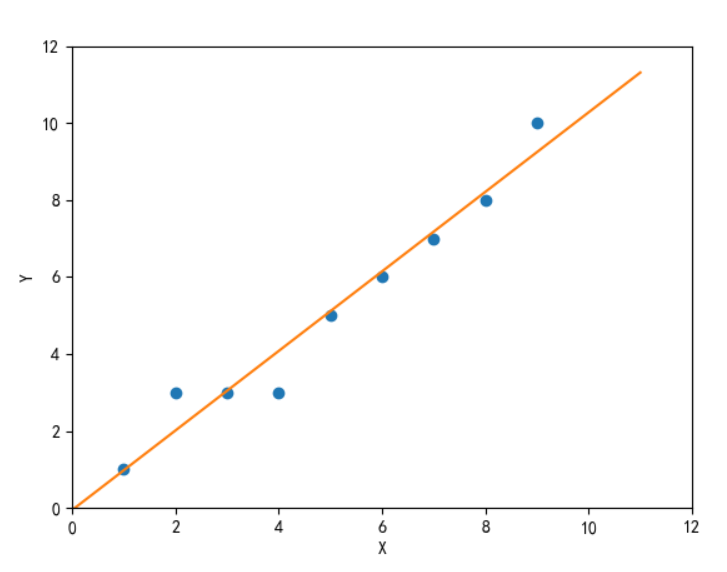展开全文• 线性回归模型建模步骤 （一元线性回归、多元线性回归）数学模型
• 对于一元线性回归模型的学习，将根据模型的学习思维进行相应的总结，具体如下。一、模型的目的通过样本数据规律来进行预测未来二、模型的公式和参数公式：其中X为自变量，Y为因变量，为残差(实际值和估计值之间的差)...
对于一元线性回归模型的学习，将根据模型的学习思维进行相应的总结，具体如下。一、模型的目的通过样本数据规律来进行预测未来二、模型的公式和参数公式：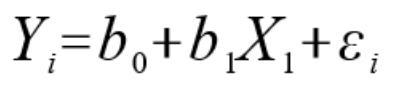其中X为自变量，Y为因变量，为残差(实际值和估计值之间的差)三、模型的解释如果X增加一个单位，在B0不变的情况下，Y增加B1个单位。如果B1为0，则Y等于B0四、模型参数的计算一元线性回归模型的计算为使用最小二乘法进行计算。其中参数的计算如下：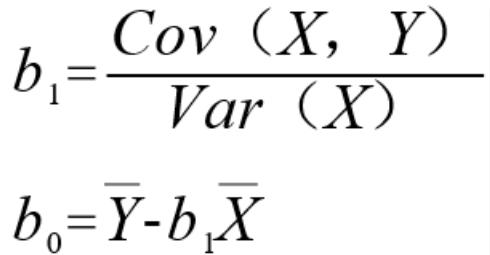考试中的涉及的计算方式：Ø 如果提供了协方差和方差，就直接按照上述公式计算。Ø 如果提供了两组数据，则使用金融计算器进行计算。第一步：2nd+7，进入数据输入页面第二步：输入X，按enter，输入Y，按enter。直到输入完成所有数据第三步：2ND+8，然后按下一个箭头，则会依次显示标准差、方差、a(为截距)、b(斜率)。五、模型的假设1. 自变量和因变量间存在线性关系。因为我们这个设置的是线性回归方程，所以要满足这个前提条件2. 自变量不随机。因为在线性方程中X和残差是不相关，同时残差项是随机数，如果自变量也是随机，那么X和残差项是相关的。3. 残差项的期望为0。因为残差项是正态分布的随机数，所以相加为0.4. 残差项的方差必须是常数。也就是残差项是稳定的，各值对应的残差的波动性为常数。如果残差项恒定，这称为同方差性，不恒定，则为异方差性。例如：前一个数据对应的残差项的方差和后一百个数据对应的残差的方差是一致的。5. 残差项与残差项之间互不关联。不能有正相关，也不能有负相关。6. 残差项是一个服从正态分布的随机数。六、模型的分析。方差分析表DF SS MSSRegression 1 RSS RSS/1Error n-2 SSE SSE/(n-2)Total n-1 TSS估计标准误：SEE=根号MSE决定系数：RSS/TSS=1-SSE/TSS,用于表示模型可以解释数据的百分比，例如0.8，则模型可以解释80%的预估数据。七、模型的假设检验第一种方法：关键值，找到关键值，看看有没有落在拒绝域中第二种方法：P值法，找到P值，看其是否小于a第三种方法：置信区间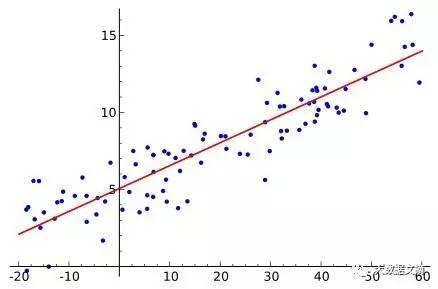展开全文• 所谓的一元线性回归其实就是找到一条直线，使得该条直线可以以最小的误差(Loss)来逼近拟合训练数据最小二乘法配合梯度下降算法，就是一个完整的线性回归过程本章知识点：1. 最小二乘法表示2. 梯度下降法原理3. 复合...
所谓的一元线性回归其实就是找到一条直线，使得该条直线可以以最小的误差(Loss)来逼近拟合训练数据最小二乘法配合梯度下降算法，就是一个完整的线性回归过程本章知识点：1. 最小二乘法表示2. 梯度下降法原理3. 复合函数求导过程下面假设我们有N个二维训练数据点，我们的预测函数为h(x) = m * x + bN个二维离散点我们希望研究这群离散点的关系，我们不妨假设其关系是线性的，那么我们需要计算出一条直线L ,使得L距离每一个离散点都很近最好的情况，我们希望所有的离散点都在L上，也就是满足L的方程，但是一条直线去拟合所有的点肯定是不现实的(不可能所有的点都满足线性关系)所以我们希望找到的L离所有的数据点尽可能的靠近，既找到每一个点(xi，yi)和L距离最小值 d = | yi - (m * xi + b)|，我们对N个d进行求和，由最小二乘法来表示误差函数为:误差函数一其中i表示第i个点，N表示样本总数，我们将Loss求和平均来作为最终的损失：误差函数二现在我们有了预测函数h(x)和损失函数Loss，那么我们怎么求出这条直线L来最好的拟合数据集呢？其实也就是求L损失函数的最小化误差，这里一般有两个方法1. 求函数极值我们把Loss看成由m和b组合的二元函数，其中x，y，i，N均是已知的，那么求Loss最小值的问题就是求函数极值为题，利用基本的高数知识，我们需要求出每个变量的偏导数，并求解这组方程组：偏导数求极值涉及简单的复合函数求导和求和求导，非常基础的高数知识，我们只要用数学知识直接解出m和b，就可以得到L的方程但是这不是一个最可靠的方法，因为计算机不适合直接求解数学方程的解，计算机是没有学过高等数学的，它只知道基本的加减乘除(本质上基于加法器实现)，而计算机的计算能力非常出众，远远超过人类，我们应该从基本的数学定理的原理出发告诉计算机做某个基本的变化，让其迭代更新，用计算量换取结果，所以我们应该利用梯度下降法去逼近真实结果2. 梯度下降法这是一个非常神奇的算法，可以说没有它，就没有现在的深度学习，几乎所有的深度学习模型都会用到梯度下降算法，如最经典的反向传播算法，对所有参数使用梯度下降算法，使得神经网络模型的各个参数在训练数据上的损失函数尽可能小，也就是模型参数最合理，再一次的让神经网络发生了质变其基本思想是：沿着损失(代价)函数梯度下降的方向搜索极小值(同理也可以沿着梯度上升的方向搜索极大值)我们设x表示方程中的变量参数，J(x)表示在给定的参数取值下，训练数据集上损失函数的大小，那么整个优化的过程可以抽象为寻找一个适合的参数x，使得J(x)最小，而目前没有一个通用的方法可以对任意损失函数直接求解最佳的参数取值，而梯度下降算法会不断迭代更新参数x，即让参数x沿着总损失很小的方向去更新梯度下降算法示意图从上图中我们看到当x = 3.5时候，J(x)取得最小值，而最低点左侧的斜率是负值，另外一侧斜率是正值，这是再好不过的收敛条件，我们只要沿着梯度的方向一点一点的下滑，下滑的过程就是求导，下滑的次数，就是迭代，而每次需要下滑多长，需要通过步长来决定，所以我们还需要定义一个步长表示每次下滑的距离一般来说，我们也将步长叫做学习率，而如何加速算法收敛速度和防止收敛过程中发生震荡，是一门值得研究的学问，有很多成熟的方法，如冲量策略，衰减因子策略等，这里不深入讲解现在定义了学习率和迭代次数，我们就可以在迭代的过程中，一边求梯度，一边下滑更新参数了很明显，我们需要在滑动中更新参数m和参数b更新参数因为梯度存在方向，所以我们使用加号并不会影响参数的更新下面用python配合numpy模拟实现梯度下降算法过程计算梯度，更新参数迭代梯度下降算法现在，我们利用梯度下降算法来优化Loss函数后，也得到了相应的m和b的值，那么也就找到了直线L = m*x + b，使得L以最小的误差去拟合训练数据一元线性回拟合结果代码和测试数据见：一元线性回归
展开全文...